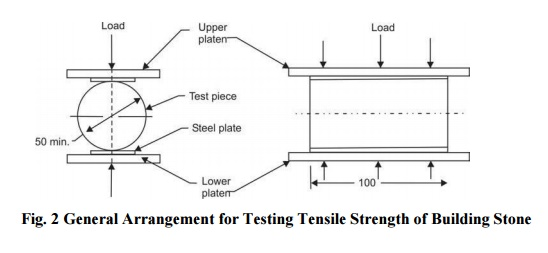Home | | Construction Materials | Tensile Strength Test (IS: 1121 (Part III))

# Tensile Strength Test (IS: 1121 (Part III))Three cylindrical test pieces of diameter not less than 50 mm and the ratio of diameter to height 1:2 are used to determine the tensile strength of the stone in each saturated (kept in water for 3 days at 20 to 30�C) and dry condition (dried in an oven at 105 � 5�C for 24 hours and cooled at room temperature).

Tensile Strength Test (IS: 1121 (Part III))

Three cylindrical test pieces of diameter not less than 50 mm and the ratio of diameter to height 1:2 are used to determine the tensile strength of the stone in each saturated (kept in water for 3 days at 20 to 30 o C) and dry condition (dried in an oven at 105 � 5 o C for 24 hours and cooled at room temperature). The general arrangement for testing tensile strength of stone is shown in Fig. 3.11. Each test piece to be tested is sandwiched in between two steel plates of width 25 mm, thickness 10 mm and length equal to the length of test piece. The load is applied without shock and increased continuously at a uniform rate until the specimen splits and no greater load is sustained. The maximum load applied to the specimen is recorded.

Split tensile strength,

S= 2W/SdL

where

S = split tensile strength (N/mm2) W = applied load (N)

d = diameter of specimen (mm), and L = length of specimen (mm)

The average of three results separately for each condition should be reported as split tensile strength of the sample. In case any test piece gives a value of as much as 15 per cent below the average, it should be examined for defects and if found defective the test piece should be rejected.

Study Material, Lecturing Notes, Assignment, Reference, Wiki description explanation, brief detail

Related Topics